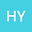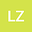The existence of nontrivial solutions for a critically coupled Schr\”{o}dinger system in a bounded domain of $\R^3$
••In this paper, we consider the following coupled Schr\”{o}dinger system with doubly critical exponents, which can be seen as a counterpart of the Brezis-Nirenberg problem $$\left\{% \begin{array}{ll} -\Delta u+\lambda_1 u=\mu_1 u^5+ \beta u^2v^3, & \hbox{x\in \Omega}, \\ -\Delta v+\lambda_2 v=\mu_2 v^5+ \beta v^2u^3, & \hbox{x\in \Omega}, \\ u=v=0,& \hbox{x\in \partial\Omega}, \\ \end{array}% \right.$$ where $\Omega$ is a ball in $\R^3,$ $-\lambda_1(\Omega)<\lambda_1,\lambda_2<-\frac14\lambda_1(\Omega)$, $\mu_1,\mu_2>0$ and $\beta>0$. Here $\lambda_1(\Omega)$ is the first eigenvalue of $-\Delta$ with Dirichlet boundary condition in $\Omega$. We show that the problem has at least one nontrivial solution for all $\beta>0$.